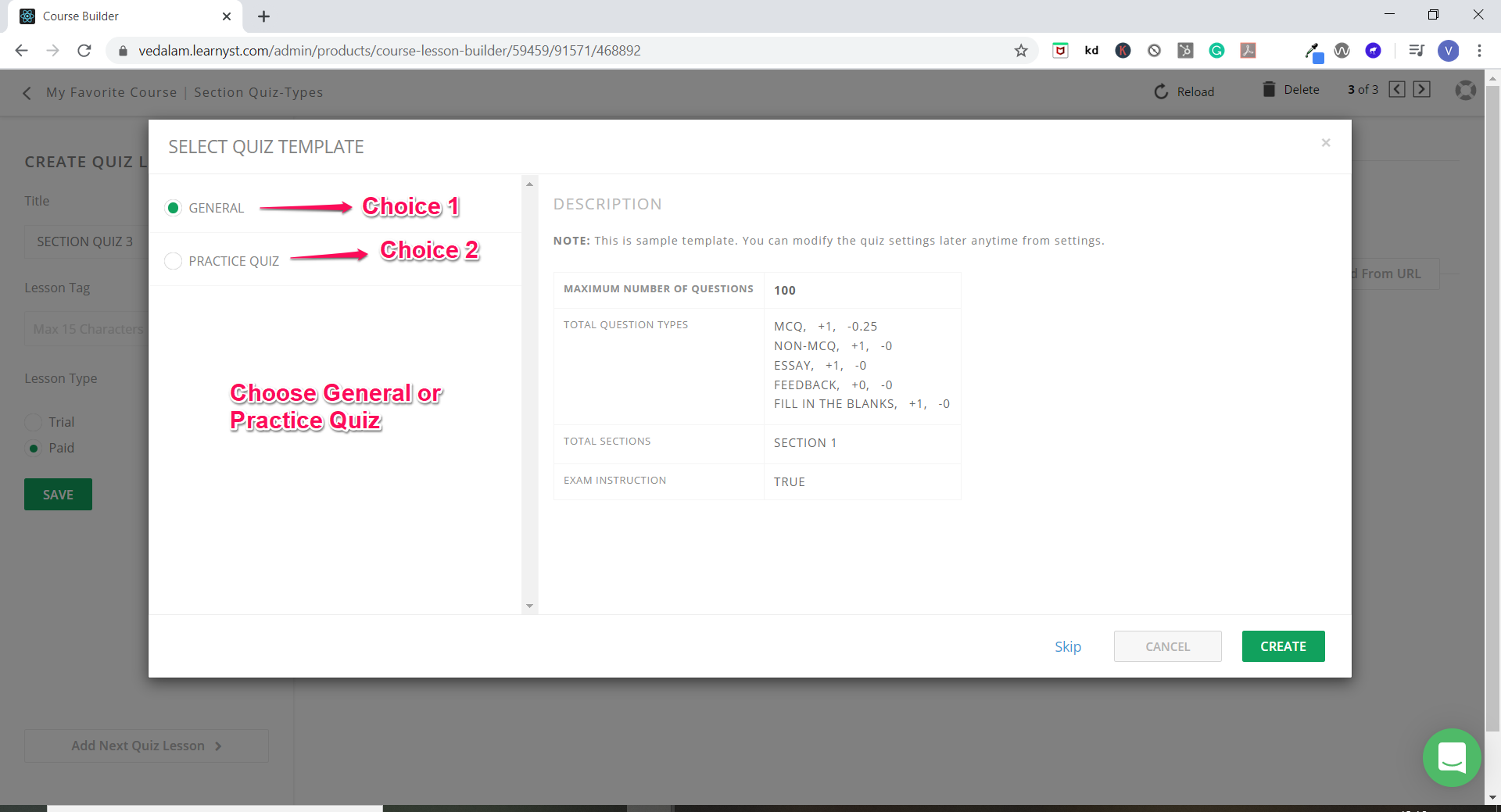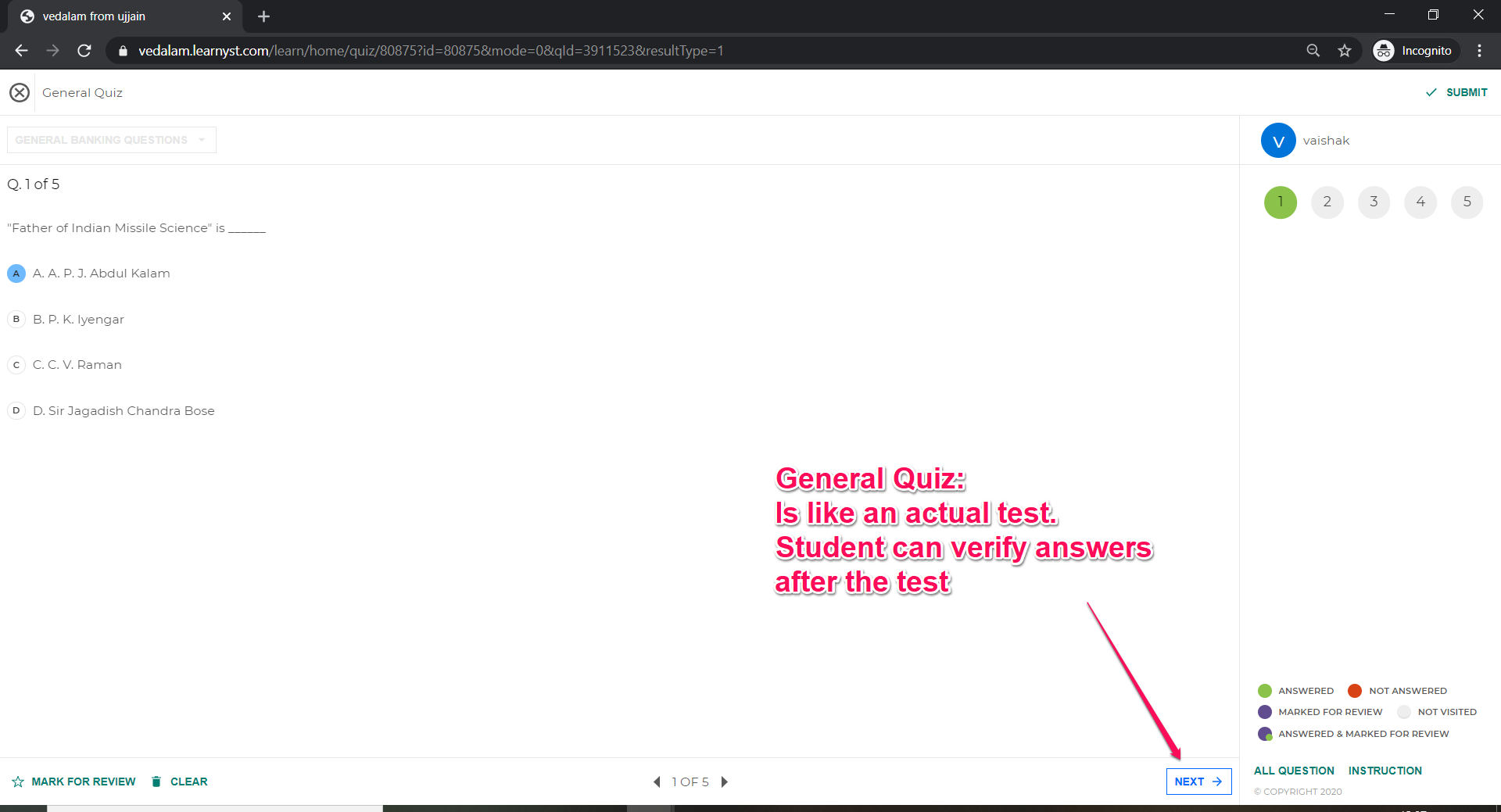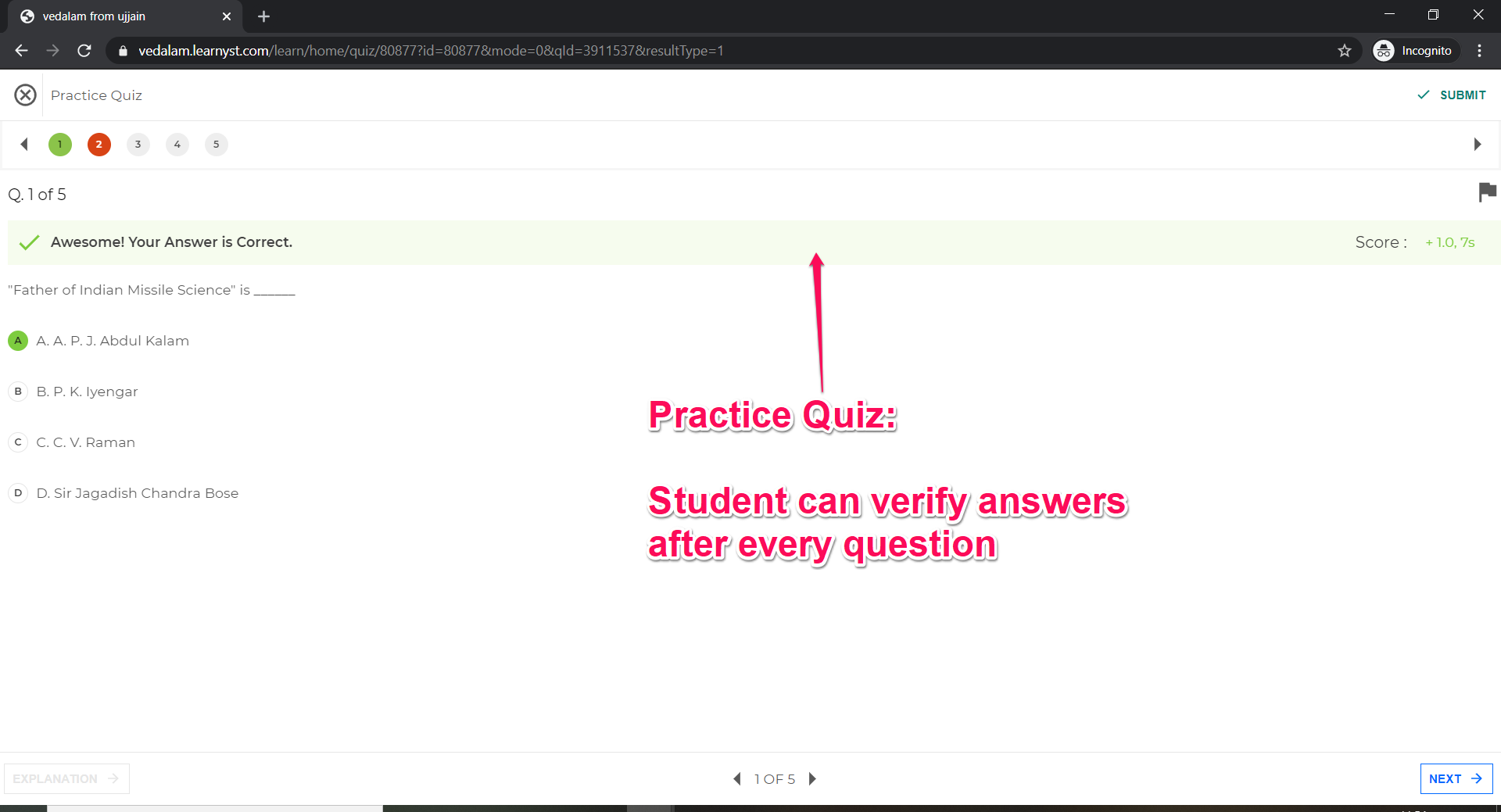Learnyst Support

# Difference between General Quiz & Practice Quiz

When you click on section quiz within your courses, you will find a screen asking you to pick a general quiz or a practice quiz.So, what are these quizzes. Lets find out.

### General Quiz

Your Students can only see the solution at the end of the test.### Practice Quiz

Gives your student a chance to verify their answers after every question.So, to summarize the only difference between these 2 quizzes is that

• Practice Quiz: Student can see solution after answering question

Practice Quiz: Student can see solution after answering question

• General Quiz: Student can see solution after completing the test.

General Quiz: Student can see solution after completing the test.

Yes, and finally the student will be able to see their performance like this graph below.